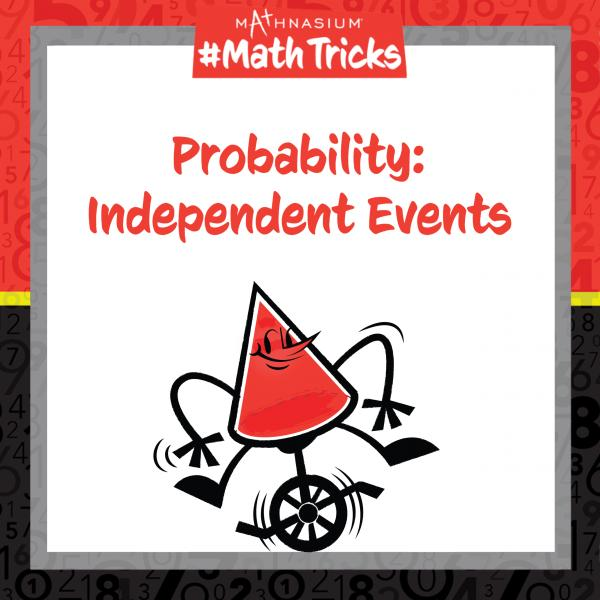# Mathnasium #MathTricks: Probability (Part 2)

By Mathnasium | Added Jan 25, 2023Welcome to Mathnasium’s Math Tricks series. Today we are calculating the probability of two independent events.

Two events are considered independent if the occurrence of one event does not affect the probability of the other event. For example, flipping a heads and flipping a tails are independent events because flipping a heads does not affect the probability of flipping a tails, and vice versa. The probability of flipping a heads or a tails will always be one-half.

In an earlier Mathnasium Math Trick we calculated basic probability by making a fraction of the number of ways an event can happen (the “favourable” outcomes) out of the total number of “possible” outcomes. To find the probability of two independent events occurring together, we multiply the probabilities of each event.

We can use a probability tree (tree diagram), or a sample space diagram, to show all possible outcomes of multiple events. A shortcut to finding the total number of combinations of a set of events is to multiply the number of possibilities for each event together, called the Fundamental Counting Principle.

Follow the examples below to find the probabilities of the two independent events.

##### Example 1: If one of four cards is picked at random and a die is rolled once, what is the probability of picking a “black card” (club or spade) or rolling more than 3?

Step 1: Identify the two independent events..
The two independent events are “picking a black card” and “rolling more than 3.”

Step 2: Find the total number of possible outcomes.
Since there are 4 cards and 6 sides on a die, there are 4 x 6 = 24 possible outcomes.

Step 3: Find the number of “winning” combinations.
The “winning” combinations contain either a “black card” or a number greater than 3:

(club, 1), (club, 2), (club, 3), (club, 4), (club, 5), (club, 6),
(heart, 4), (heart, 5), (heart, 6),
(diamond, 4), (diamond, 5), (diamond, 6).

There are 18 “winning” combinations.

Step 4: Calculate the probability.
The probability of picking a “black card” or rolling a number greater than 3 is 18/24 or 3/4.

##### Example 2: A spinner has 3 options, and a bag has 2 red marbles, 3 blue marbles, and 1 green marble. What is the probability of not pulling a red marble and not spinning a 1?

Step 1: Identify the two independent events.
The two independent events are “not pulling a red marble” (so, pulling a blue or green marble) and “not spinning a 1” (so, spinning a 2 or 3).

Step 2: Find the total number of possible outcomes.
Since there are 3 spinner options and 6 marbles, there are 3 x 6 = 18 possible outcomes.

Step 3: Find the probability of each independent event.
Recall there are 3 blue marbles and 1 green marble.
The probability of pulling a blue or green marble is 4/6 or 2/3, and the probability of spinning a 2 or a 3 is 2/3.

Step 4: Calculate the probability.
To find the probability of not pulling a red marble and not spinning a 1 we multiply the probabilities of each event: 2/3 x 2/3 = 4/9.